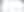# Lanas.

## Digital craftsmanship for the solar industry.self.__wrap_b=(t,n,e)=>{e=e||document.querySelector(`[data-br="\${t}"]`);let s=e.parentElement,r=B=>e.style.maxWidth=B+"px";e.style.maxWidth="";let o=s.clientWidth,u=s.clientHeight,a=o/2-.25,c=o+.5,p;if(o){for(r(a),a=Math.max(e.scrollWidth,a);a+1<c;)p=Math.round((a+c)/2),r(p),s.clientHeight===u?c=p:a=p;r(c*n+o*(1-n))}e.__wrap_o||(typeof ResizeObserver!="undefined"?(e.__wrap_o=new ResizeObserver(()=>{self.__wrap_b(0,+e.dataset.brr,e)})).observe(s): false&&0)};self.__wrap_b(":R4ba:",1)

A cool little Ecommerce Store which is built on the Shopify Storefront API and Nextjs.

The Shopify Dashboard looks like this: